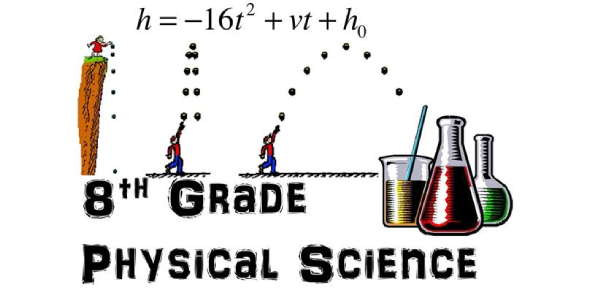# 8th Grade Physical Science Quiz: Test

23 Questions | Total Attempts: 924SettingsAre you familiar with 8th-grade physical science? Would you like to try this quiz? In 8th grade, students learn about the scientific method and science terminology. Physical science topics include magnetism and electricity, heat and light, forces in liquids and gases, electrical and nuclear energy, Newton’s law of motion, and the periodic table of elements. If you want to learn more about 8th-grade physical science, this quiz can be helpful to you.

• 1.
Which of the following is a physical property of an element?
• A.

Color

• B.

Ability to Rust

• C.

Flammability

• D.

Ability to Tarnish

• 2.
During a chemical change, the form of a substance is altered, but not its identity.
• A.

True

• B.

False

• 3.
A(n)____ is a group of atoms that are joined together by chemical bonds.
• 4.
Which of the following is NOT a charactereistic of a compound?
• A.

Has different properties from the elements that formed it.

• B.

Pure substance made of two or more elements.

• C.

Different samples have different properties.

• D.

Can be represented by a chemical formula.

• 5.
A change in matter in which energy is taken in is an endothermic change.
• A.

True

• B.

False

• 6.
A measure of the average energy of random motion of particles of matter is______________
• 7.
Anything that has mass and takes up space is an example of
• A.

Energy

• B.

Chemical change

• C.

Matter

• D.

Temperature

• 8.
Every chemical or physical change in matter includes a change in...
• A.

Volume

• B.

Temperature

• C.

Energy

• D.

Mass

• 9.
A(n) _____ of a pure substance can be observed without changing the substance.
• A.

Chemical property

• B.

Atom

• C.

Element

• D.

Physical property

• 10.
Electrical energy is the energy of electrically charged particles moving from one place to another.
• A.

True

• B.

False

• 11.
A chemical bond is
• A.

A group of atoms that are joined together.

• B.

The basic particle of matter.

• C.

The force that holds two atoms together.

• D.

A substance formed from the chemical combination of two or more atoms.

• 12.
Which of the following is an example of a chemical change?
• A.

Melting butter

• B.

Mixing milk and chocolate syrup.

• C.

Breaking glass

• D.

Burning fuel

• 13.
Breaking down water into the elements of hydrogen and oxygen is an example of a chemical change.
• A.

True

• B.

False

• 14.
______ is the ability to do work or cause change.
• 15.
Which of the following is NOT true of atoms?
• A.

They are composed of molecules.

• B.

They can combine with other atoms.

• C.

They make up elements.

• D.

They are extremely small.

• 16.
In science lab, this chemical, which is also a pure element, turned color when it reacted with some of the crime scene suspect chemicals.
• 17.
Ice is an example of the liquid state of matter.
• A.

True

• B.

False

• 18.
In the CSICT crime scene lab, this liquid acid was an example of a chemical change by producing bubbles when it reacted with some of the powder from the suspects.
• 19.
In the diagram below, "A" represents a pure substance or a mixture?
• 20.
In the diagram below does "B" represents a pure substance or a mixture?
• 21.
In the diagram below, does "C" represent a pure substance or a mixture?
• 22.
In the diagram below, does "D" represent a pure substance or a mixture?
• 23.
IS AN ELEMENT OR COMPOUND REPRESENTED IN FIGURE "D" beIow?
Related TopicsBack to top